Courses

# RD Sharma Solutions -Ex-7.3, Decimals, Class 6, Maths Class 6 Notes | EduRev

## RD Sharma Solutions for Class 6 Mathematics

Created by: Abhishek Kapoor

## Class 6 : RD Sharma Solutions -Ex-7.3, Decimals, Class 6, Maths Class 6 Notes | EduRev

The document RD Sharma Solutions -Ex-7.3, Decimals, Class 6, Maths Class 6 Notes | EduRev is a part of the Class 6 Course RD Sharma Solutions for Class 6 Mathematics.
All you need of Class 6 at this link: Class 6

Question 1

i) Five hundred twenty five and forty hundredths

Solution: 525+40100 = 525.40

ii) Twelve and thirty five thousandths

Solution: 12+351000 = 12.035

iii) Fifteen and seventeen thousandths

Solution: 15+171000 = 15.017

iv) Eighty eight and forty eight hundredths

Solution: 88+48100 = 88.48

Question 2

i) 137+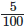Solution: We have 1 hundred, 3 tens , 7 ones and 5 hundredths. Therefore , the decimal is 137.05

ii) 20+9+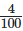Solution: We have 2 tens, 9 ones and 4 hundredths. Therefore , the decimal os 29.04

Question 3

i)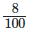Solution: We have 8 hundredths. Therefore , the decimal is 0.08

ii)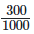Solution: In its lowest form , the fraction is 310. We have 3 tenths. Therefore , the decimal is 0.3

iii)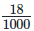Solution: We have eighteen thousandths. Therefore the decimal is 0.018

iv)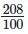Solution: 208100=200100+8100=2+8100

We have 2 and 8 hundredths. Therefore , the decimal is 2.08

1.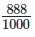Solution: 8881000=8001000+801000+81000=810+8100+81000

We have 8 tenths, 8 hundredths and 8 thousandths. Therefore , the decimal is 0.888

Question 4

i)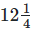Solution: 1214=12+14

=12+254(25)=12+25100 =12.25

ii) 718 = 7+18

Solution: 7+1(1258)(125) = 7+1251000 = 7.125

iii) 5120 = 5+120

Solution: 5+1(520)(5) = 5+510 = 5.05

Question 5

i) 0.04

Solution:
= 0+0.04

= 0+4 hundredths

= 0+4100

=125

ii)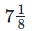Solution:
2.34

= 2+0.34

= 2+34 hundredths

=2+34100

=2(100100) + 34100

= 200100+34100

= 234100

= 11750

iii)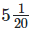0.342

= 0+342 thousandths

= 3421000

= 171500

iv) 1.20

= 1+0.20

= 1+20hundredths

= 1+20100

= 100100+20100

= 120100

= 65

v) 17.38

= 17+0.38

= 17+38 hundredths

= 17+38100

= 17(100100) +38100

= 1700100+38100

= 1738100

= 86950

Question 6

i) Here, we have 2 tens , 9 ones, 4 tenths and 1 hundredths. Therefore , the decimal is
Solution: 29.41

ii) Here, we have 3 tens, we have3 tens, 4 tenths , 8 hundredths and 3 thousandths. therefore , the decimal is
Solution: 30.483

iii) Here, we have 1 hundred, 3 tens, 7 ones and 5 hundredths. Therefore , the decimal is
Solution: 137.05

iv) Here, we have 7 tenths , 6 hundredths and 4 thousandths . therefore , the decimal is
Solution: 0.764

v) Here, we have 2 tens, 3 ones, 2 tenths and 6 thousandths. Therefore , the decimal is
Solution: 23.206

vi) Here, we have 7 hundreds, 2 tens, 5 ones and 9 hundredths. therefore , the decimal is
Solution: 725.09

103 docs

,

,

,

,

,

,

,

,

,

,

,

,

,

,

,

,

,

,

,

,

,

,

,

,

,

,

,

,

,

,

;